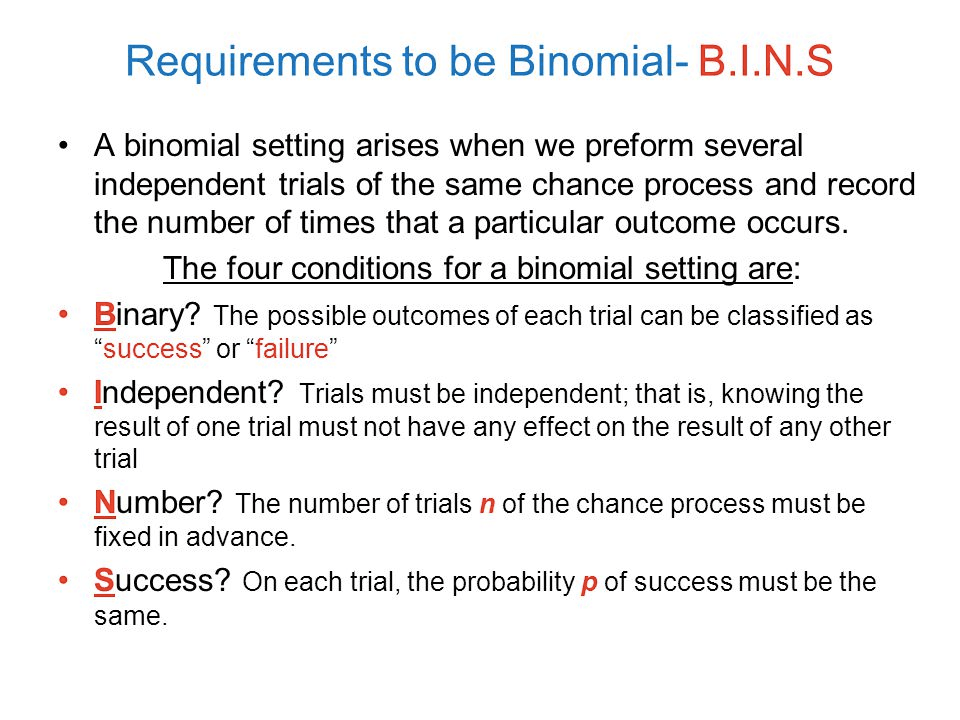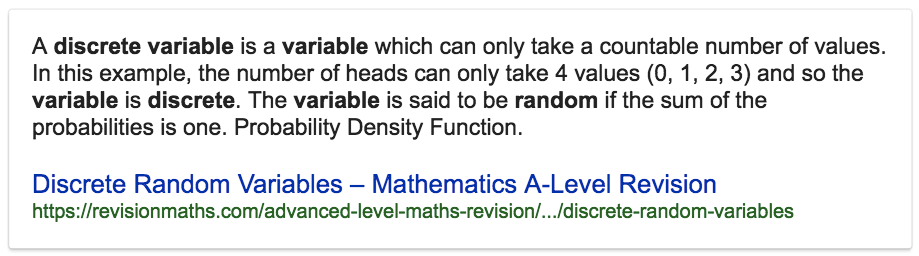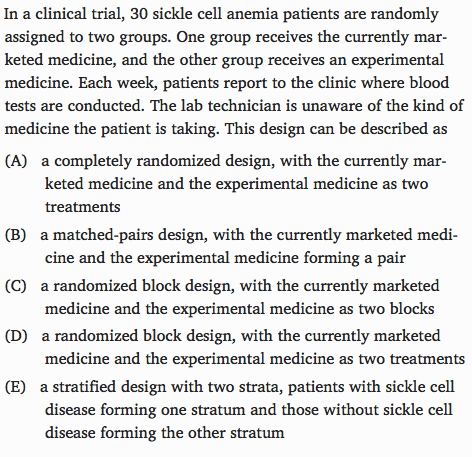# Question 2• We cannot use a binomial model unless we know that the probability of drawing a woman for the pool is nearly constant.

• However, since we are drawing 12 jurors without replacement, this is not necessarily true unless the jury pool is very large (at least 120)# Question 11

• A discrete variable takes only a countable number of values. The number of test questions a student guesses the answers to is a random variable with possible values 0, 1, 2,…n, where n is the number of questions on the test.# Question 29

• If we take different samples from the same population, the estimates from the different samples will be different. The difference in percentages may be entirely due to sampling variation.# Question 30• This experiment consists of two treatments, the currently marketed medicine and the experimental medicine. Patients are not matched, and no blocks are formed. Only patients with sickle cell disease are involved in the experiment.

# Question 31

• Confidence intervals are constructed as Statistic ± Margin of Error.

• Therefore, the statistic is always right in the center of the confidence interval.